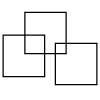#### You may also like### Three Squares

What is the greatest number of squares you can make by overlapping three squares?### Two Dice

Find all the numbers that can be made by adding the dots on two dice.### Biscuit Decorations

Andrew decorated 20 biscuits to take to a party. He lined them up and put icing on every second biscuit and different decorations on other biscuits. How many biscuits weren't decorated?

# Domino Sorting

##### Age 5 to 7Challenge Level

Natasha and Izzy from Finney Primary School say:

Our solution was that we gave each domino a number going left to right (and top to bottom) . The first domino 1, second 2 and so on. The first solution was the odd numbers (in order 1, 2 so on) were:
odd: 1,4,5,6. even: 2,3,7,8,9.

So, in other words the dominoes which have an odd number of spots are:And the rest have an even number of spots. Natasha and Izzy say that none of the dominoes was left over. Rachel from Brynteg School explains that:

All whole numbers are even or odd. The numbers on the dominoes are even or odd so there are no numbers left.

Jack and Peter answered the second part of the problem. They wrote:

For the pairs equalling five you have the 0:3 + 0:2, 0:1 + 1:3, 1:1 + 1:2. The ones left over are, 2:2, 2:3 and 3:3.
Other ways include, 2:2 + 0:1, 1:1 + 0:3, 0:2 + 1:2 then all the others are left over.
There are always some dominoes left over but there are two that are always left over: 3:3 and 3:2 because they both equal five or over.

Well done, Jack and Peter. There are other ways to make pairs which total 5 and Thomas from Reading explains why:

You could pair the dominoes up to total a number of five spots in three ways at any one time by pairing a three with a two or a one with a four. There are two dominoes which add up to two and they could each go with either one of the dominoes which add up to three. There are also two dominoes with a total of four spots and either one of those could go with the domino with only one spot on it.

So here are all the possible ways of grouping the dominoes to make 5:

2,2 and 0,1 0,3 and 0,2 1,2 and 1,1 or
3,1 and 0,1 0,3 and 0,2 1,2 and 1,1 or
2,2 and 0,1 0,3 and 1,1 1,2 and 0,2 or
3,1 and 0,1 0,3 and 1,1 1,2 and 0,2